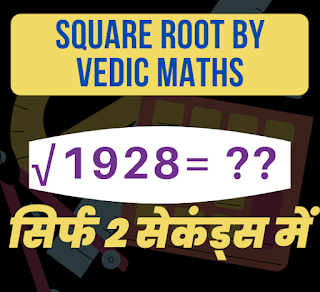# Easy to learn Vedic Mathematics square root tips & trick.Square Root Tricks Using Vedic Maths

# How To Find Square Root Easily Using Vedic Math Tricks for Govt Exam?

In most of the Government Exams, the Quantitative Aptitude on Numerical Ability section will often test your speed. You need to be quick with calculations if you want to ace Banking & SSC Exams. As in these examinations calculation based questions are often asked. Here in this blog, you will know how to find the square root of a number.

### Some Important Point for Square Roots Math tricks :

• Squares of numbers from 1 to 9 are 1, 4, 9, 16, 25, 36, 49, 64, 81, and 100.
• Square of a number cannot end with 2, 3, 7, and 8. OR number ending with 2 , 3, 7 and 8 cannot have perfect squareroot.
• Squareroot of a number ending with 1 (1, 81) ends with either 1 or 9 (10’s compliment of each other).
• Squareroot of a number ending with 4 (4, 64) ends with either 2 or 8 (10’s compliment of each other).
• Squareroot of a number ending with 9 (9, 49) ends with either 3 or 7 (10’s compliment of each other).
• Squareroot of a number ending with 6 (16, 36) ends with either 4 or 6 (10’s compliment of each other).
• If number is of ‘n’ digits then square root will be ‘n/2’ OR ‘(n+1)/2’ digits.

Example:

Square root of 1296

1. The number ends with 6, Since it’s a perfect square, square root will end with 4 or 6.
2. Need to find 2 perfect squares between which 12 exists.
Numbers are 16(42) and 9(32).
3. 16 is more than 12 so, the perfect square will be a 34 or 36.
4. Now find the square of 35, which is 1225
5. 1225 is smaller than 1296.
6. Hence the square root of 1296 is = 36.

•I bring to you the BEST for students of Class 9th - 12th. I (Balkishan Agrawal) aim at providing complete preparation for CBSE Board Exams (Maths) along with several other competitive examinations like NTSE, NSO, NSEJS, PRMO, etc. & Maths Reasoning, Vedic Maths at the school level. Wishing you great learning in all aspects of your life!

error: Content is protected !!